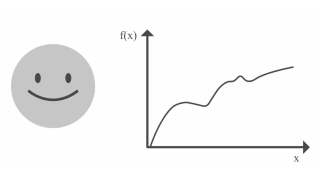# Understanding Kalman Filters, Part 5: Nonlinear State Estimators8 min
Beginner
Video
Theory

This video explains the basic concepts behind nonlinear state estimators, including extended Kalman filters, unscented Kalman filters, and particle filters.

A Kalman filter is only defined for linear systems. If you have a nonlinear system and want to estimate system states, you need to use a nonlinear state estimator. This video explores different nonlinear filters to help you choose the one that will work for your nonlinear system. Extended Kalman filters linearize the distribution around the mean of the current estimate and then use this linearization in the predict and update states of the Kalman filter algorithm. An unscented Kalman filter selects a minimal set of sample points (also referred to as sigma points) from the Gaussian distribution, and it propagates them through the nonlinear system. It then computes the mean and covariance of the new set of transformed sample points and uses these to find the new state estimate. The working principles behind particle filters are similar to unscented Kalman filters, but particle filters can approximate any arbitrary distribution. For this, particle filters require a larger set of points (referred to as particles).

This resource is included in the following topics and journeys: